# How to Convert Weekday Name to Number in Excel?

• Last Updated : 24 Jun, 2022

MS-Excel is a program that is part of the Microsoft Office suite. It’s a multi-row, multi-column electronic spreadsheet that’s used to organize data, visualize data, and do various calculations/operations. Each row and column comprise a cell, each sheet contains 1048576 rows and 16383 columns. Each cell has an address that is determined by the column name and row number, such as A1, D2, and so on. A cell reference is another name for this.

Dates can be converted and formatted in various ways. Sometimes we need weekdays to be in integer format and sometimes in string format. According to the use case, we will need to convert from string to number and number to string. We can use MATCH() or LOOKUP() worksheet functions for this purpose. We have to map each weekday to the number pattern we want. For example: if we want ‘Monday’ to be mapped to 1, ‘Tuesday’ to 2, and so on. We might also like to start the week with Sunday, then Sunday will be mapped or converted to 1, Monday to 2, and so on…

### Using MATCH Function

In this approach, we use the MATCH function to convert weekday names to numbers in Excel. Let us discuss this with the help of the below example. In the below example column A contains weekday names and column B has the converted integers for each weekday. To do this task we use the MATCH() function. This function is used to get the relative position of the given element from a range of the cell.

Syntax:

MATCH  (lookup_value, lookup_array, [match_type])

Where,

loopup_value = This is a mandatory argument. Lookup value The value for which you are looking.
lookup_array= This is a mandatory argument. Lookup array The array of data to be searched.
[match_type]= This is an optional argument. Match type The following values can be used: 1, 0, -1. 1 is the default value.

• 1 – The MATCH method looks for the greatest value less than or equal to Lookup value.
• 0 – The MATCH function will look for the first value that matches Lookup value exactly.
• -1 – The Match function will look for the least number greater or equal to Lookup value.

In the below example the match function takes the lookup value from the ‘A’ column, then an array of week names is passed in the lookup_array, and the lookup_value is checked in the lookup array. As the look-up array started from Monday, MATCH() function for Monday results in 1 and also because match_type is 0, which will look for the first value that matches the lookup value exactly. Finally, the weekdays are converted to numbers in column B of our spreadsheet. Let us discuss them step by step:

Step 1: All the weekday names are given in the A column of our excel sheet.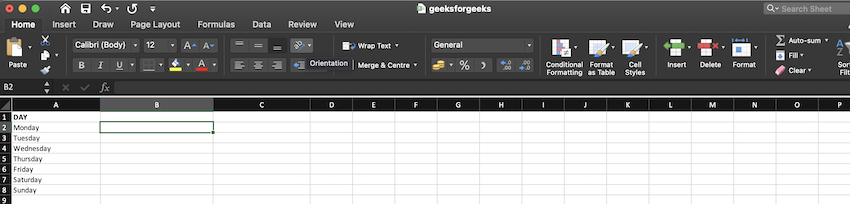Step 2: In the match function, the lookup value is taken as A2, and the lookup array is given as {“Monday”,”Tuesday”,”Wednesday”,”Thursday”,”Friday”,”Saturday”,”Sunday”}, match type is given as 0 as we need an exact match.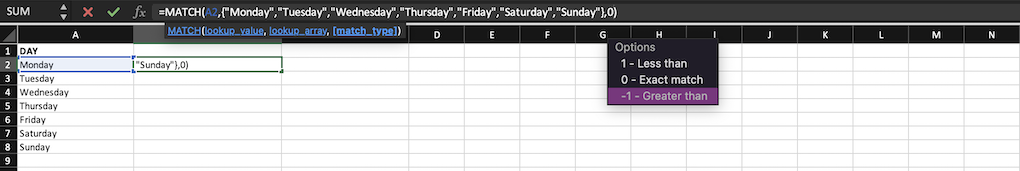Step 3: After using the formula, in the B2 cell we get the weekday number as 1 for the weekday ‘Monday’.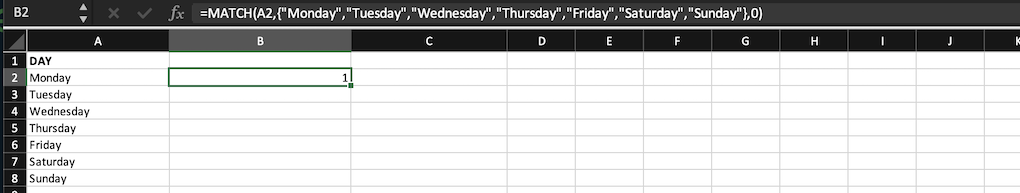Step 4: By dragging the cell, we get converted/ matched values(Numbers) for all the weekday names of column A in column B.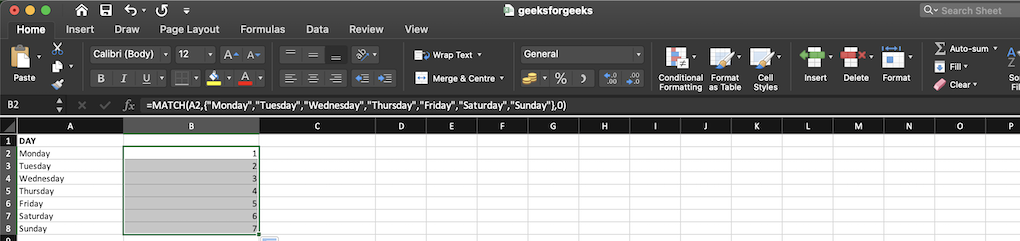Here as the lookup_array started from “Sunday”, Sunday is converted to 1, and the rest follows.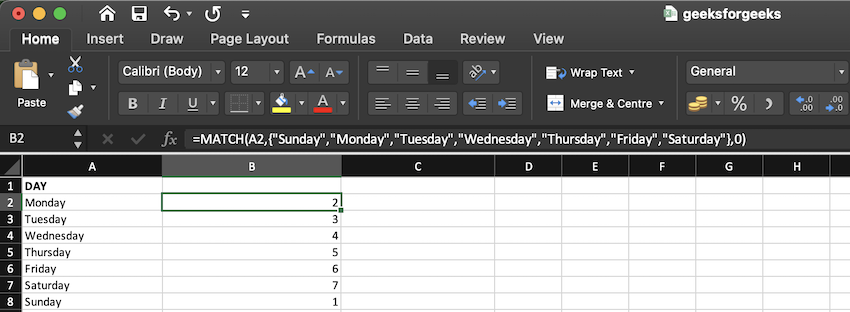### Using LOOKUP Function

Instead of using the MATCH() function, we can also use the LOOKUP() function. This function is used to find a matching lookup either in a row or column range and return the corresponding value from another row or column range.

Syntax:

= LOOKUP (lookup_value, lookup_vector, [result_vector])

Where,

lookup_value: This is a mandatory argument. Lookup value ,A value in the lookup vector that you want to find.

lookup_vector: This is a mandatory argument. Lookup vector, A range in Excel that just has one row or column.

Result vector: This input is optional. A range in Excel that just has one row or column. Lookup vector must be the same size. The lookup function searches the lookup value range for a value and returns it from the same point in the result vector.

In this example, we use the LOOKUP() function. A1 is given as the lookup_value, and a lookup_vector is given which has all the weekdays and mappings. In column B we use this function to convert the weekday name to a number in the first cell and then we drag to apply the same function to all the cells. Using the match() function is a better approach. Let us discuss them step by step:

Step 1: Weekday names are given in column A of the excel sheet.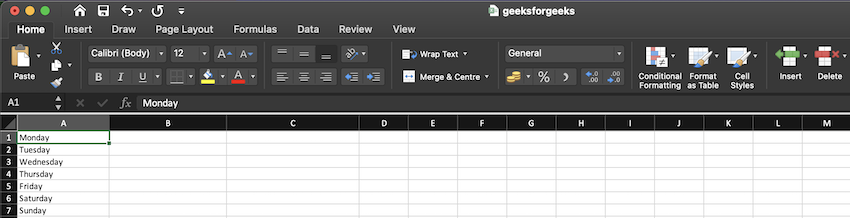Step 2: The lookup function is applied on the b1 cell of the column, look up value is given as A1 and the lookup array is ,{“Friday”,5;”Monday”,1;”Saturday”,6;”Sunday”,7;”Thursday”,4;”Tues  day”,2;”Wednesday”,3} .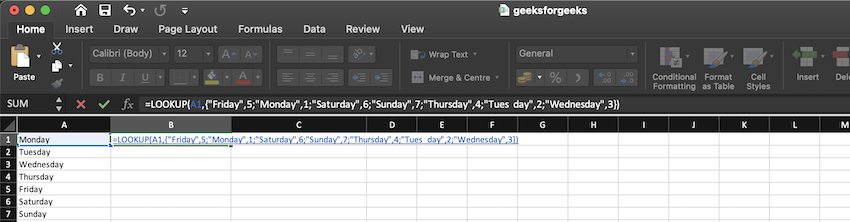Step 3: After using the function, the weekday name in cell A1 is changed to a weekday number in cell B1.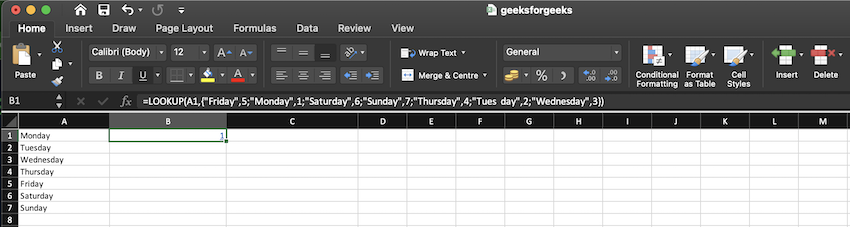Step 4: We drag the cell, to apply the function to the whole column and the result is shown in column B.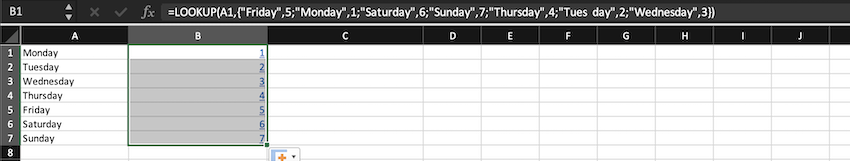My Personal Notes arrow_drop_up
Recommended Articles
Page :